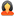Question

All Subjects Physics General Physics Physics Multiple Problems

Offered Price \$ 18.00

Physics Multiple Problems

Question # 00006319
Subject: Physics
Topic: General Physics
Due on: 01/09/2014
Posted On: 01/08/2014 11:54 PM

Rating:
4.1/5Posted ByQuestions:
15716
Tutorials:
15379
Feedback Score:

Question

1. A completely submerged object always displaces

A.) weight of fluid equal to its weight B.) volume of fluid equal to its volume. C.) density of fluid equal to its density. D.) all of these. E.) none of these

2. Which of these is not true about the buoyant force.

A.) It is equal to the weight of fluid displaced by a submerged object. B.) It is present in both liquids and gases. C.) It is equal to the volume of fluid displaced by the submerged object D.) It always acts upwards against the force of gravity

3. Two liquid columns of the same height, one of water, the other of mercury, exert pressures on the fluid at the bottom of the columns. Which of these statements is true?

A.) The pressure exerted by the column of mercury is 13.6 times greater than that of water B.) The pressure exerted by the column of mercury is 13.6 times less than that of water C.) The pressures exerted by both fluids are equal D.) None of the above

4. According to Charles’ Law, if the temperature of a gas is increased by a factor of 2, its volume A.) increases by a factor of 2 B.) increases by a factor of 4, C.) becomes half of its original volume D.) does not change E.) none of the above

5. A 24kg piece of metal displaces 4L of water when submerged. What is its density?

a) 4000 kg/m3 b) 2000 kg/m3 c) 1000 kg/m3 d) 6000 kg/m3 e) 1500 kg/m3

6. What is the product of the reaction between carbon dioxide and water?

Carbonic Acid

7. Why does a solution of a strong acid conduct electricity better than a solution of weak acid having the same concentration?

8. The source of all magnetism is a) tiny bits of iron b) small lodestones c) the motion of electrons d) none of the above

9. The primary of a transformer connected to 120V has 100 turns. The secondary has 10 turns. What is the output voltage (i.e. the voltage from the secondary)? A) 12V B)15V C) 30V D) 40V E) 25V

10. When you thrust a bar magnet to and fro into a coil of wire, you induce A) direct current B) alternating current C) neither of the above D) increase the resistance of the wire

11. I) What is a neutralization reaction? II) Name a strong acid and a weak acid.

12. I) Name three ways an element can be oxidized. II) In the reaction represented below, hydrogen sulfide , H2S, burns in the presence of oxygen, O2, to produce water H2O and sulfur dioxide, SO2:

2H2S + 3O2 2H2O + 2SO2. In this reaction, is sulfur oxidized or reduced?

13. I) What is a circuit? II) Identify 2 differences between a series and a parallel circuit. III) Three lamps with resistances 1 ?, 2 ? and 3 ? are connected in series in a circuit with a voltage source of 12V. A) What is the current in the circuit? B) Calculate the voltage drop across each lamp. (Please show your work)

Tutorials for this Question
Available for
\$ 18.00

Physics Multiple Problems Solution

Tutorial # 00006051
Posted On: 01/08/2014 11:59 PM
Posted By:expert-mustangQuestions:
15716
Tutorials:
15379
Feedback Score:
Tutorial Preview … has 10 turns. What is the output voltage (i.e. the voltage from the secondary)? A) …
* - Additional Paypal / Transaction Handling Fee (3.9% of Tutorial price + \$0.30) applicable